Summing Across Multiple Sheets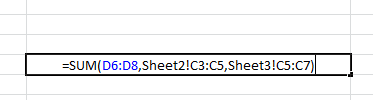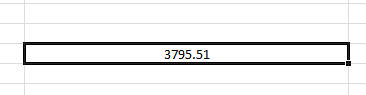In this article, we will learn How to sum across different sheets in Excel.

Scenario:

Sometimes we need to access different values from different worksheets of the same excel book. Here we are accessing it to add multiple cells in Excel 2016. In this article, we will understand how to sum the values located on different sheets in excel 2016. We will use the SUM function to add numbers. SUM function allows you to sum values anywhere in the sheet. You can access it by typing or via selecting values from the sheet.

SUM Formula in Excel

SUM function adds up the values.

SUM = number 1 + number 2 + …

Syntax:

 =SUM(list1, sheet_name1! list2, sheet_name2! list3, ...)

list1 : list of values to sum from the same sheet where function is used.

sheet_name1! list2 : list of values to sum from separate sheet.

sheet_name2! list3 : another list of values to sum from a separate sheet.

Example :

All of these might be confusing to understand. Let's understand how to use the function using an example. Here we have a list of values from sheet1, sheet2 and sheet3.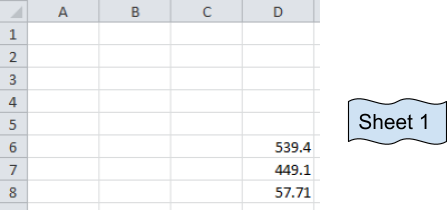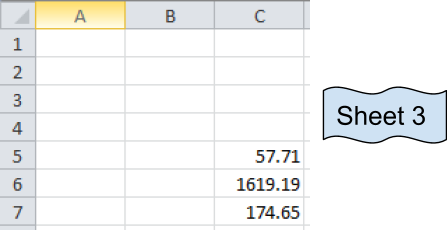These are numbers from three different sheets and desired output sum will be in Sheet 1.

Now we use the SUM function

Formula:

 =SUM(D6:D8, Sheet2!C3:C5,Sheet3!C5:C7)

Explanation:

The resulting output is in Sheet 1.

D6:D8 adds the values of Sheet 1  D6+D7+D8

C3:C5 adds the values of Sheet 2  C3+C4+C5

C5:C7 adds the values of Sheet 3   C5+C6+C7.

It's basically the addition of values in cells

D6+D7+D8 + C3+C4+C5 + C5+C6+C7

You can select the cells separated by commas to add the numbers.Your formula will look like the above image.

Press Enter and your desired sum will be here in Sheet 1.As we can see, the Sum function returns the sum. You can use the Autosum option in the Home tab under Editing section.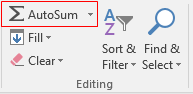Click the arrow key for more options like shown below.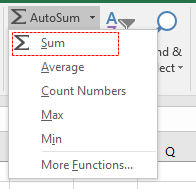Then select the cells to add up values in Excel. You can sum across the rows and columns using the SUM function.

Here are all the observational notes using the formula in Excel
Notes :

1. Text values and empty cells are ignored. The formula works with numbers only.
2. The function returns error if any cell contains error value.
3. SUM function can access upto 255 different arrays separated by commas. But there is no limit to the number of cells.

Hope this article about How to sum across sheets in Excel is explanatory. Find more articles on calculating values and related Excel formulas here. If you liked our blogs, share it with your friends on Facebook. And also you can follow us on Twitter and Facebook. We would love to hear from you, do let us know how we can improve, complement or innovate our work and make it better for you. Write to us at info@exceltip.com.

Related Articles :

All About Excel Named Ranges : excel ranges that are tagged with names are easy to use in excel formulas. Learn all about it here.

The Name Box in Excel : Excel Name Box is nothing but a small display area on top left of excel sheet that shows the name of active cell or ranges in excel. You can rename a cell or array for references.

How to Get Sheet name of worksheet in Excel : CELL Function in Excel gets you the information regarding any worksheet like col, contents, filename, ..etc. Learn how to get the sheet name using the CELL function here.

How To Get Sequential Row Number in Excel : Sometimes we need to get a sequential row number in a table, it can be for a serial number or anything else. In this article, we will learn how to number rows in excel from the start of data.

Increment a number in a text string in excel : If you have a large list of items and you need to increase the last number of the text of the old text in excel, you will need help from the two TEXT and RIGHT functions.

Popular Articles :

How to use the IF Function in Excel : The IF statement in Excel checks the condition and returns a specific value if the condition is TRUE or returns another specific value if FALSE.

How to use the VLOOKUP Function in Excel : This is one of the most used and popular functions of excel that is used to lookup value from different ranges and sheets.

How to use the SUMIF Function in Excel : This is another dashboard essential function. This helps you sum up values on specific conditions.

How to use the COUNTIF Function in Excel : Count values with conditions using this amazing function. You don't need to filter your data to count specific values. Countif function is essential to prepare your dashboard.

Terms and Conditions of use

The applications/code on this site are distributed as is and without warranties or liability. In no event shall the owner of the copyrights, or the authors of the applications/code be liable for any loss of profit, any problems or any damage resulting from the use or evaluation of the applications/code.